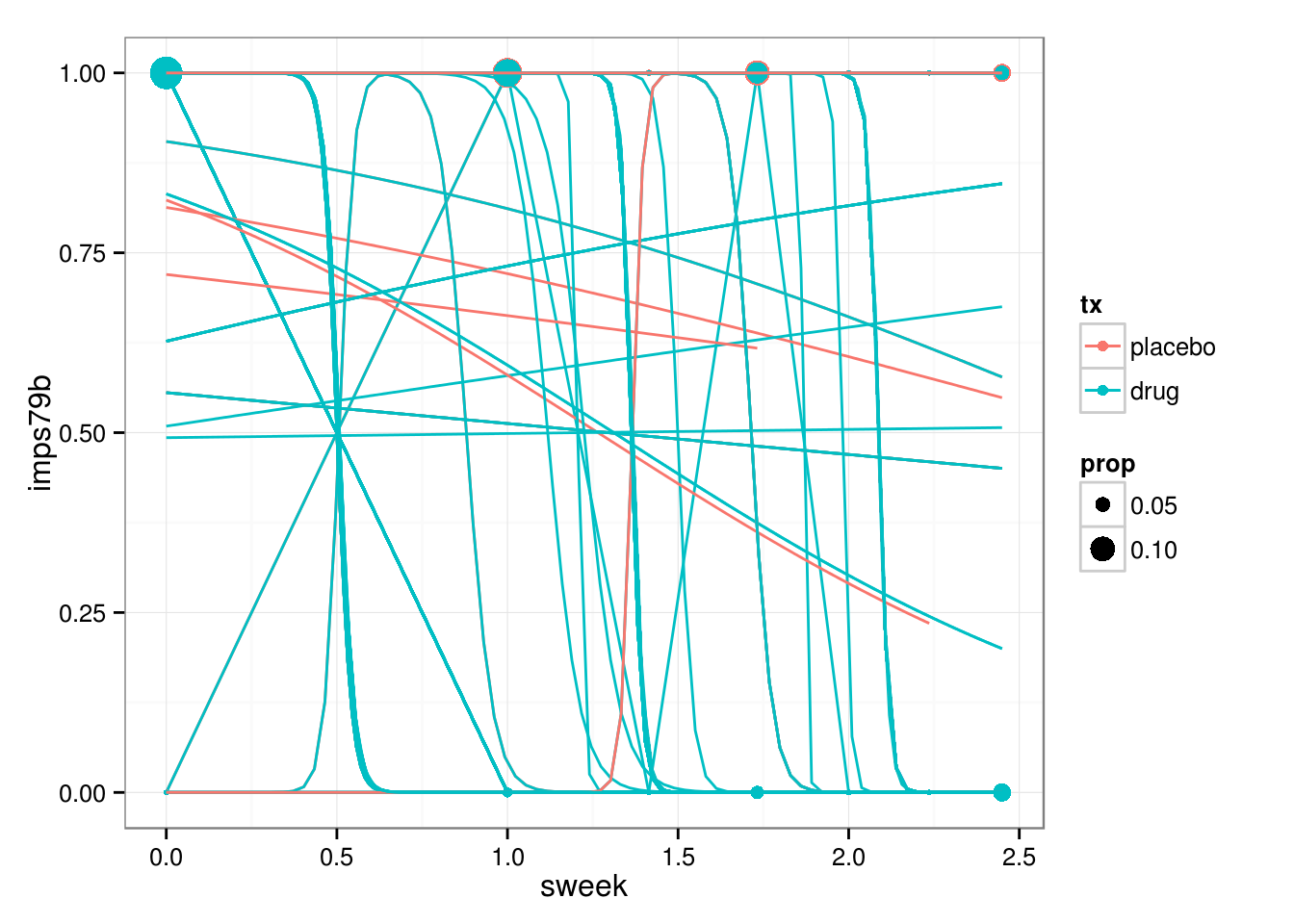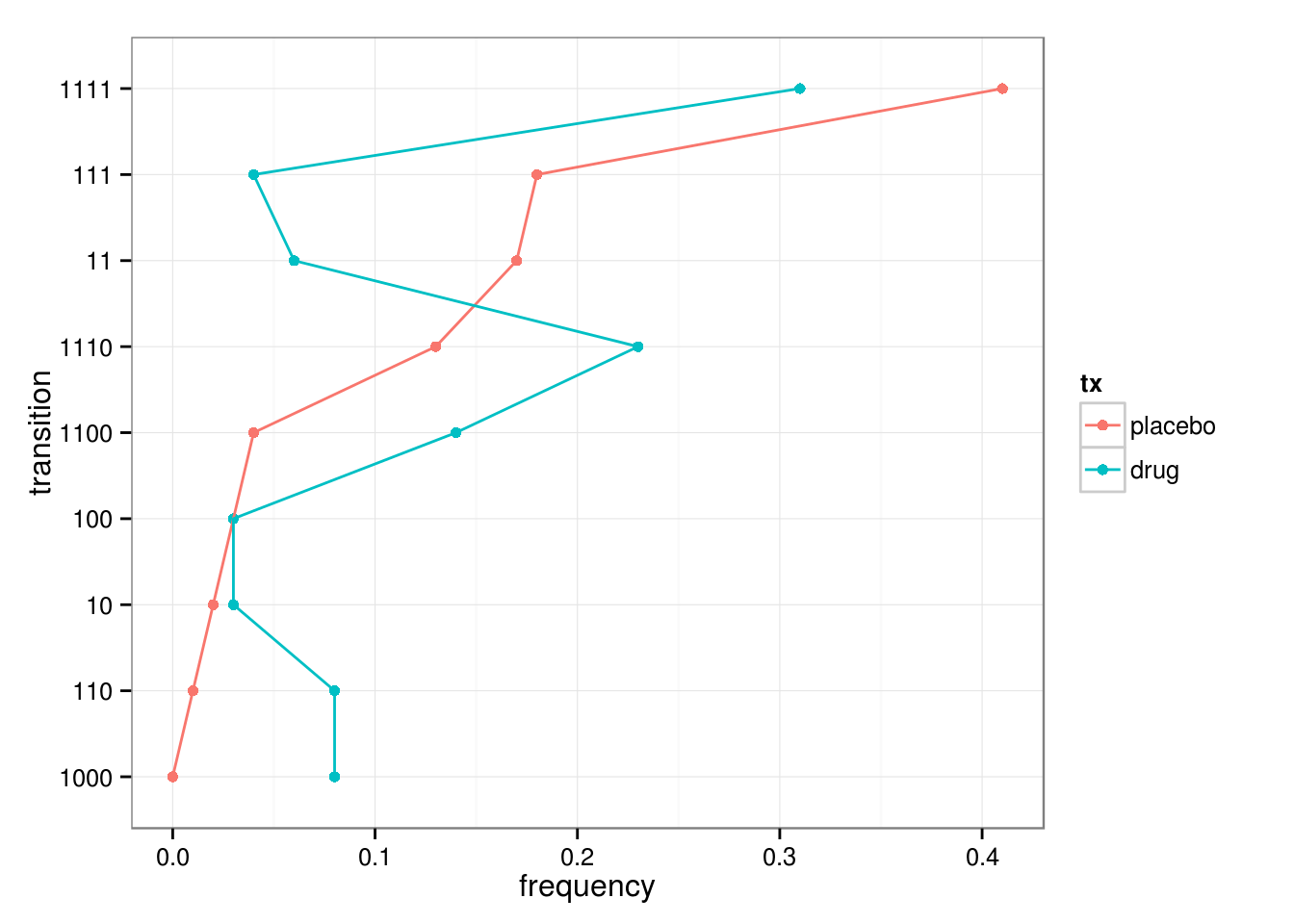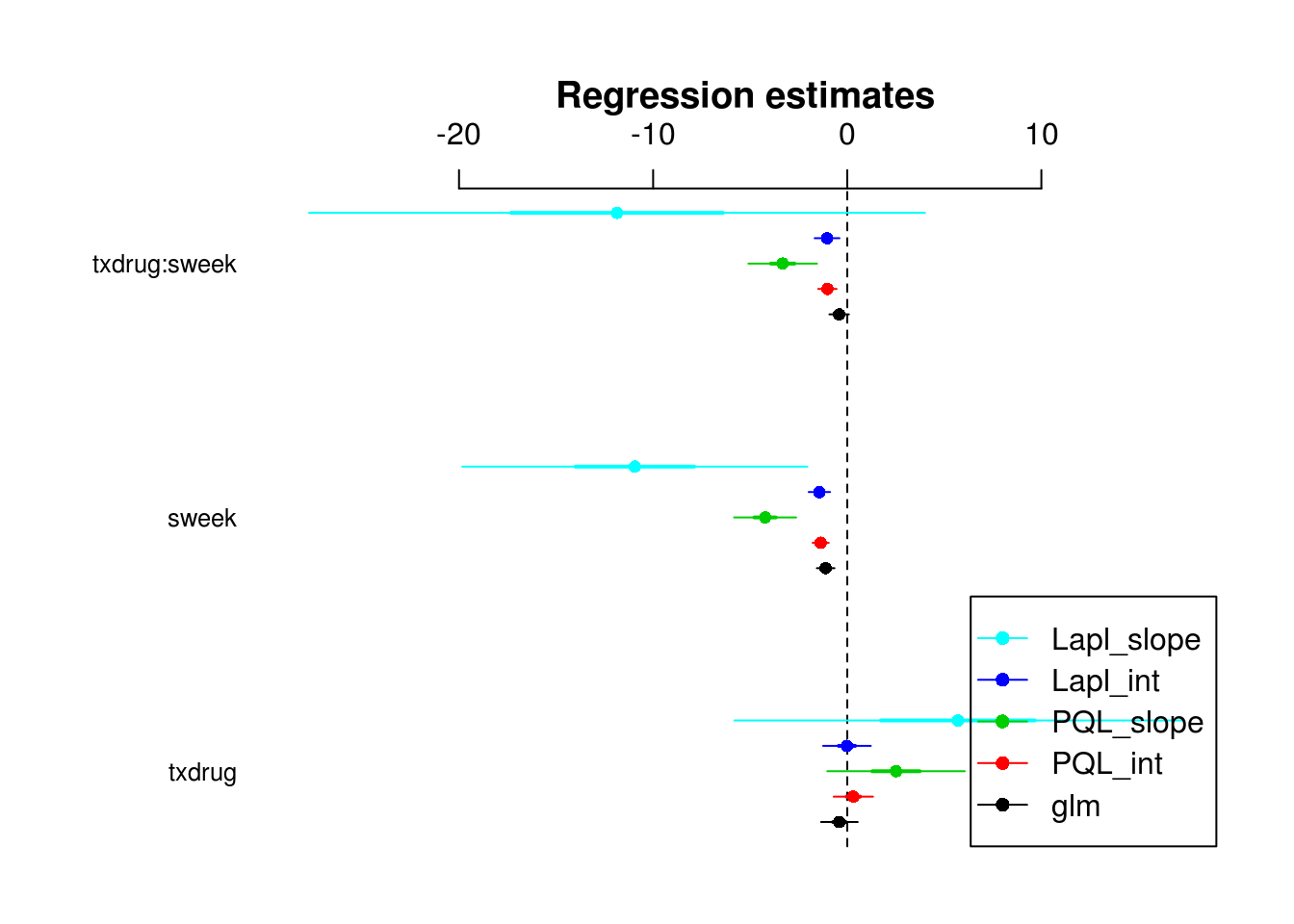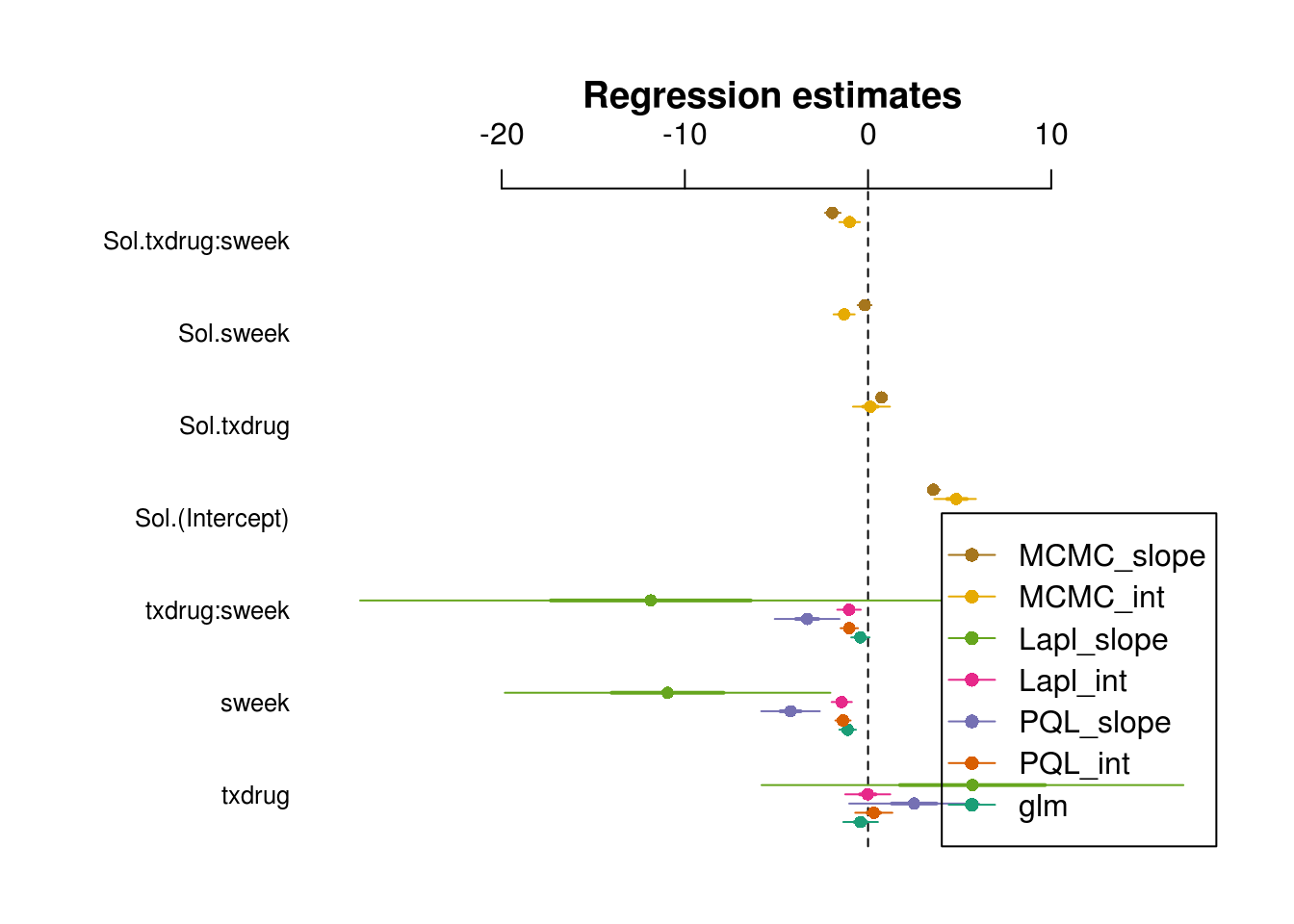## Exploring GLMMs for schizophrenia data

Data from here, inspiration from Hedeker’s web page

fn <- "schizx1.dat"
if (!file.exists(fn)) {
}

(This almost works: I found an extra Ctrl-Z character at the end of the file that I had to hand-edit out …)

dd0 <- read.table("schizx1.dat")
names(dd0) <- c("id","imps79","imps79b","imps79o","int","tx",
"week","sweek","txswk")
apply(dd0,2,function(x) sum(x==-9,na.rm=TRUE))
##      id  imps79 imps79b imps79o     int      tx    week   sweek   txswk
##       0    1456    1456    1456       0       0       0       0       0

(I could have used na.strings=c("-9","NA") but that seems dangerous in general – what if there are legitimate -9 values in some columns?)

Utility functions/packages:

na.vals <- function(x,crit) {
x[crit] <- NA
return(x)
}
library(plyr)  ## for mutate()
library(MASS)  ## for glmmPQL
library(nlme)
library(lme4)
library(reshape2) ## melt()
library(MCMCglmm)
library(ggplot2); theme_set(theme_bw())
## get this with repos="http://www.math.mcmaster.ca/bolker/R":
library(coefplot2)
library(RColorBrewer)
cvec <- brewer.pal(7,"Dark2")

Post-process data a little bit (this follows the steps on Hedeker’s web page):

dd <- mutate(dd0,
imps79=na.vals(imps79,imps79<0),
imps79b=na.vals(imps79b,imps79b<0),
imps79b=factor(imps79b,labels=c("le mild","ge moderate")),
tx=factor(tx,labels=c("placebo","drug")))

The sweek variable is square-root of week (presumably done to improve linearity on the logit scale??)

ggplot(dd,aes(sweek,imps79b,colour=tx,group=id))+geom_line()+
stat_sum(aes(group=tx))+
geom_smooth(method="glm",family="binomial",se=FALSE)Trying to facet on individuals is slow & silly (most of the space is taken up by the facet labels; we could strip the plots down to sparklines and remove the labels, but I’m not sure how much it would help).

(Not evaluated:)

ggplot(dd,aes(sweek,imps79b,colour=tx,group=id))+geom_line()+
facet_wrap(~id)

Try sparklines-style plots, possibly in batches? (473 individuals is a big challenge for anything other than spaghetti plots …)

Check experimental design:

with(dd,table(tx,sweek))
##          sweek
## tx          0   1 1.4142 1.7321   2 2.2361 2.4495
##   placebo 108 108    108    108 108    108    108
##   drug    329 329    329    329 329    329    329
with(na.omit(dd),table(tx,sweek))
##          sweek
## tx          0   1 1.4142 1.7321   2 2.2361 2.4495
##   placebo 107 105      5     87   2      2     70
##   drug    327 321      9    287   9      7    265

Try reducing the data to sequences: most data are observed at only 4 unique time periods

dd$rweek <- round(dd$sweek^2)
dd2 <- na.omit(subset(dd,rweek %in% c(0,1,3,6)))
nrow(na.omit(dd))-nrow(dd2)  ## lose only 34 cases (out of 1600)
##  34
dd3 <- ddply(dd2,"id",summarise,
tx=tx,
trans=paste(imps79b,collapse=""))
ttab <- with(dd3,table(trans,tx))
ttab0 <- ttab[rowSums(ttab)>10,]
fracrep <- c(sum(ttab0),length(unique(dd\$id)))

After stripping out the unusual sequences, we still have 408 out of 437 individuals represented:

##       tx
## trans  placebo drug
##   10         2    9
##   100        3   10
##   1000       0   25
##   11        17   20
##   110        1   25
##   1100       4   42
##   111       18   13
##   1110      13   70
##   1111      41   95

Or normalize by column total (= number of individuals in the treatment) and sort by frequency in placebo arm:It might be a special-case method, but I can imagine that we could come up with a statistical model (e.g. a Markov model/ finite-state machine?) for the likelihood of these sequences under an appropriate distribution of individual-level REs …

Fit regular GLM, PQL with random-intercept and random-slopes models, Laplace with random intercept:

m0 <- glm(imps79b~tx*sweek,dd,family=binomial)
m1 <- MASS::glmmPQL(imps79b~tx*sweek,random=~1|id,dd,family=binomial,
verbose=FALSE)
m2 <- MASS::glmmPQL(imps79b~tx*sweek,random=~1+sweek|id,dd,family=binomial,
verbose=FALSE)
m3 <- glmer(imps79b~tx*sweek+(1|id),dd,family=binomial)

Try Laplace with random slopes. Extremely slow: lots of ‘step-halving’ action, and eventually fails to converge with maxgrad=180.4 (!!)

m4 <- glmer(imps79b~tx*sweek+(1+sweek|id),dd,family=binomial)
## Warning: Model failed to converge with max|grad| = 180.415 (tol = 0.001,
## component 4)

Try both models with MCMCglmm:

## random intercept
m5 <- MCMCglmm(imps79b~tx*sweek,
random=~id,
data=na.omit(dd),
family="categorical",verbose=FALSE)
## random intercept+slope
m6 <- MCMCglmm(imps79b~tx*sweek,
random=~us(sweek):id,
data=na.omit(dd),
family="categorical",verbose=FALSE)
cbind(glm=coef(m0),glmmPQL_int=fixef(m1),
glmmPQL_slope=fixef(m2),lme4_int=fixef(m3),
lme4_slope=fixef(m4))
##                     glm glmmPQL_int glmmPQL_slope    lme4_int lme4_slope
## (Intercept)   3.7027053   4.6846075     12.113539  5.29432838  41.553451
## txdrug       -0.4054238   0.3149482      2.513707 -0.01603148   5.701931
## sweek        -1.1125529  -1.3674879     -4.231725 -1.43552801 -10.942024
## txdrug:sweek -0.4179643  -1.0223856     -3.325614 -1.03903393 -11.860041
coefplot2(list(glm=m0,PQL_int=m1,PQL_slope=m2,
Lapl_int=m3,Lapl_slope=m4),
legend=TRUE)Needs more work to combine m5 properly:

coefplot2(list(glm=m0,PQL_int=m1,PQL_slope=m2,
Lapl_int=m3,Lapl_slope=m4,
MCMC_int=m5,MCMC_slope=m6),
col=cvec,
legend=TRUE)